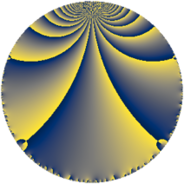# Properties

 Label 49.4.eLevel $49$ Weight $4$ Character orbit 49.e Rep. character $\chi_{49}(8,\cdot)$ Character field $\Q(\zeta_{7})$ Dimension $78$ Newform subspaces $1$ Sturm bound $18$ Trace bound $0$

# Related objects

## Defining parameters

 Level: $$N$$ $$=$$ $$49 = 7^{2}$$ Weight: $$k$$ $$=$$ $$4$$ Character orbit: $$[\chi]$$ $$=$$ 49.e (of order $$7$$ and degree $$6$$) Character conductor: $$\operatorname{cond}(\chi)$$ $$=$$ $$49$$ Character field: $$\Q(\zeta_{7})$$ Newform subspaces: $$1$$ Sturm bound: $$18$$ Trace bound: $$0$$

## Dimensions

The following table gives the dimensions of various subspaces of $$M_{4}(49, [\chi])$$.

Total New Old
Modular forms 90 90 0
Cusp forms 78 78 0
Eisenstein series 12 12 0

## Trace form

 $$78 q - 5 q^{2} - 5 q^{3} - 53 q^{4} - 23 q^{5} + 19 q^{6} - 31 q^{8} - 174 q^{9} + O(q^{10})$$ $$78 q - 5 q^{2} - 5 q^{3} - 53 q^{4} - 23 q^{5} + 19 q^{6} - 31 q^{8} - 174 q^{9} + 9 q^{10} - 103 q^{11} + 364 q^{12} - 35 q^{13} + 161 q^{14} - 245 q^{15} - 205 q^{16} - 285 q^{17} + 16 q^{18} + 628 q^{19} + 553 q^{20} - 21 q^{21} - 605 q^{22} + 149 q^{23} + 653 q^{24} - 370 q^{25} - 511 q^{26} - 65 q^{27} + 70 q^{28} - 187 q^{29} + 84 q^{30} + 1276 q^{31} + 1399 q^{32} - 23 q^{33} - 765 q^{34} - 805 q^{35} - 1691 q^{36} - 1531 q^{37} - 1041 q^{38} - 1351 q^{39} - 1759 q^{40} - 301 q^{41} + 3395 q^{42} - 257 q^{43} - 883 q^{44} + 3105 q^{45} + 1593 q^{46} + 733 q^{47} - 1948 q^{48} + 1288 q^{49} + 6148 q^{50} + 1197 q^{51} - 1099 q^{52} - 285 q^{53} + 660 q^{54} + 2641 q^{55} - 1988 q^{56} - 2352 q^{57} + 1173 q^{58} - 3603 q^{59} - 175 q^{60} - 2613 q^{61} - 1927 q^{62} - 3066 q^{63} + 1589 q^{64} - 371 q^{65} - 2175 q^{66} + 352 q^{67} + 6076 q^{68} + 5549 q^{69} - 6293 q^{70} - 2623 q^{71} + 6220 q^{72} + 2039 q^{73} - 2411 q^{74} - 3903 q^{75} + 4130 q^{76} + 1029 q^{77} - 3759 q^{78} + 44 q^{79} - 1608 q^{80} + 1394 q^{81} - 10920 q^{82} - 553 q^{83} - 7798 q^{84} + 497 q^{85} - 2985 q^{86} - 4273 q^{87} - 2197 q^{88} - 3957 q^{89} - 2958 q^{90} + 14119 q^{91} - 9136 q^{92} + 6272 q^{93} + 14912 q^{94} + 5866 q^{95} + 21882 q^{96} - 1540 q^{97} - 2303 q^{98} + 10768 q^{99} + O(q^{100})$$

## Decomposition of $$S_{4}^{\mathrm{new}}(49, [\chi])$$ into newform subspaces

Label Dim $A$ Field CM Traces $q$-expansion
$a_{2}$ $a_{3}$ $a_{5}$ $a_{7}$
49.4.e.a $78$ $2.891$ None $$-5$$ $$-5$$ $$-23$$ $$0$$